Next: YACC - Yet Another Compiler Up: Parsing Previous: Non-recursive implementation of predictive parsing

## LL(1) Grammars

LL(1) GRAMMARS AND LANGUAGES. A context-free grammar G = (VT, VN, S, P) whose parsing table has no multiple entries is said to be LL(1). In the name LL(1),

• the first L stands for scanning the input from left to right,
• the second L stands for producing a leftmost derivation,
• and the 1 stands for using one input symbol of lookahead at each step to make parsing action decision.
A language is said to be LL(1) if it can be generated by a LL(1) grmmar. It can be shown that LL(1) grammars are
• not ambiguous and
• not left-recursive.
Moreover we have the following theorem to characterize LL(1) grammars and show their importance in practice.

Theorem 3   A context-free grammar G = (VT, VN, S, P) is LL(1) if and if only if for every nonterminal A and every strings of symbols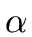,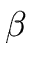such that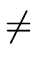and A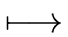|we have
1. FIRST(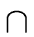FIRST()  =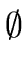,
2. if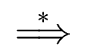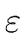then FIRST(FOLLOW(A)  =.

LL(K) PARSING TABLES. The notion of FIRST sets can be generalized to describe the first k tokens of a string (with k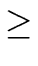1). This leads to LL(k) parsing tables where

• the rows are labelled by non-terminals and
• we have a column for every sequence of k terminals.
This is rarely done in practice since these tables are so large.

LL(K) GRAMMARS. Grammars parsable with LL(k) parsing tables are called LL(k) grammars. The LL(k) grammars have the following properties.

• If G is a LL(k) grammar then G is also a LL(k + 1) grammar (for every k1).
• For every k1, if G is a LL(k) grammar the G is not ambiguous.

Remark 7   Here are some practical observations on the use of LL(1) grammars and predictive parsing.
• The main difficulty in using predictive parsing is in writing a LL(1) grammar for the source language.
• The parser of a compiler often uses
• predictive parsing methods for control constructs and
• bottom-up parsing methods for expressions.Next: YACC - Yet Another Compiler Up: Parsing Previous: Non-recursive implementation of predictive parsing
Marc Moreno Maza
2004-12-02